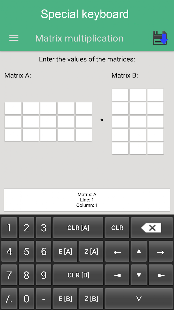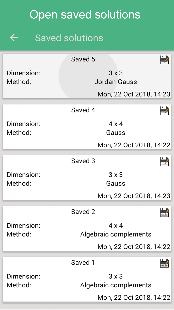The following operations are available in the application:
– Solution of systems of linear equations by methods:
★ Gauss;
★ Cramer;
★ Jordan-Gauss;
★ The inverse matrix;
– Finding of the matrix determinant by methods:
★ Sarrus (only for the matrix 3×3);
★ Decomposition on the first line;
★ Reduction to the triangular form;
– Finding of inverse matrix by methods:
★ Gauss;
★ Jordan-Gauss;
★ Algebraic Supplements;
– Solution of matrix equations
– Matrix construction
– Multiplication of a matrix by a number
– Finding the rank of the matrix
– Transposition of matrices
– Matrix multiplication
– Subtraction of matrices

Application Features
– Special keyboard for more convenient data entry;
– Full, step by step description of solutions;
– Ability to save decisions;
– Ability to edit saved solutions

Keywords: matrix calculator,
matrix operations, matrix determinant,
matrix calculator, linear equations

What’s New:
– Optimization. Now the application runs a little faster and more stable.
– Added ability to swap matrices
– Added the ability to copy the matrix and paste them in the right place. This allows you to quickly and easily move the matrix between methods.

Screenshots

••••••••